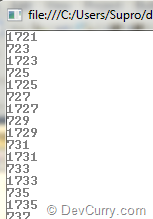# LINQ: Generate Odd Numbers using Parallel Execution

 Tweet
A couple of months ago, I had written on Generate Odd Numbers within a Range using LINQ. In that post, I had demoed how to ‘sequentially’ generate odd numbers within a Range. However what if you have to generate a large set of numbers and are not interesting in generating the numbers in a sequence, you can use Parallel Execution. The ParallelEnumerable.Range() is just the right method for this requirement which generates a parallel sequence of integer numbers. Let’s see an example:

```static void Main(string[] args)
{
IEnumerable<int> oddNums
= ((ParallelQuery<int>)ParallelEnumerable.Range(20, 2000))
.Where(x => x % 2 != 0)
.Select(i => i);

foreach (int n in oddNums) { Console.WriteLine(n); }
}```

The code more or less remains the same as demoed in my previous article. However there’s an important observation to make - the cast to a ParallelQuery<int>. It is this casting that creates a parallel execution instead of a sequential one.

Run the application, and as you can see, the odd numbers are generated parallel, in no particular oder.